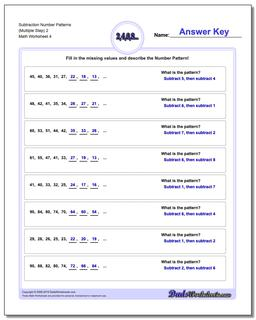# Math Worksheets: Number Patterns: Number Patterns: Subtraction Number Patterns (Multiple Step) 2 (Fourth Worksheet)## Subtraction Number Patterns (Multiple Step) 2 (Fourth Worksheet)

PropertyValue
DescriptionSubtraction Number Patterns (Multiple Step) 2: Number pattern problems using only subtraction operations. (Fourth Worksheet)
Resource TypeWorksheet